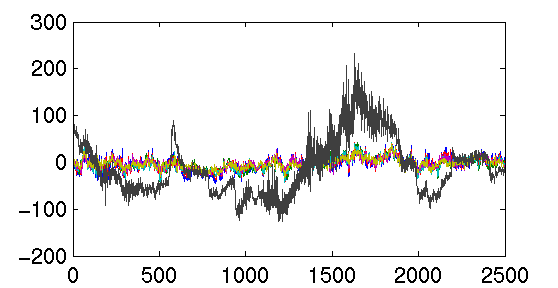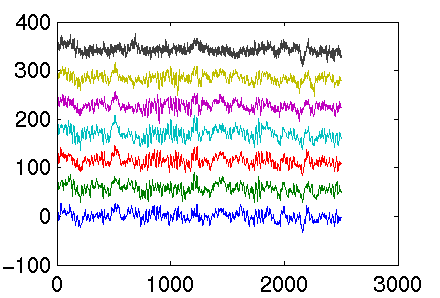##Getting Started with Matlab for EEG Experiments
Chuck Anderson

This page continues to grow as new material is added to show new and current project members how to use the software tools we are developing.

# Setting Up Matlab

Create a subdirectory in your home directory named matlab. In this subdirectory, create the file `startup.m` with the contents
```format compact
more off
```
When matlab starts, it evaluates the lines in this file, as if you had typed them in interactively. This adds some of my matlab directories to your matlab path.

You must also add the matlab/bin directory to your shell's search path. For csh, you can add these lines to your `.cshrc` file:

```# for Matlab
setenv MATLAB /s/parsons/l/sys/matlab64
alias matlabwho \$MATLAB/etc/lmstat -a
setenv PATH \${PATH}:\${MATLAB}/bin
setenv LD_LIBRARY_PATH  \${LD_LIBRARY_PATH}:\${MATLAB}/extern/lib/sol2:\${MATLAB}/extern/lib/glnx86
setenv MANPATH  \${MANPATH}:\${MATLAB}/man
setenv JAVA_HOME /usr/local/java
```

After sourcing your `.cshrc` file or re-logging in, you can start matlab:

```> matlab -nojvm

< M A T L A B >
Version 6.1.0.450 Release 12.1
May 18 2001

To get started, type one of these: helpwin, helpdesk, or demo.
For product information, visit www.mathworks.com.

Diary is being appended to DiaryMatlab
>>
```
I like running matlab with the `-nojvm` option, so I don't waste time starting up the Java virtual machine and I also don't get the Java-based GUI for interacting with matlab. I prefer the old text-based form.

The final thing you see is `>>`, the matlab prompt. Type `quit` and return to leave matlab.

# Retrieve Our EEG Data

Your first task is to load some of the EEG data we have available. Do so with the matlab function `load_eegdata`:
```>> load_eegdata
eegdata_subjects =
's1'    's3'    's4'    's5'    's6'
'rest'    'letter'    'math'    'rotation'    'counting'
'aeo'    'lceo'    'maeo'    'reo'    'veo'
eegdata_trials =
1     2     3     4     5     6     7     8     9    10
eegdata_rows =
'c3'    'c4'    'p3'    'p4'    'o1'    'o2'    'eog'
eegdata_example =
eegdata{2,3,4}(1:6,:) returns a 6x2500 matrix of the EEG channels
for subject 2 (s3), task 3 (math), and trial 4
```
You can see where this function is located by
```>> which load_eegdata
```
so you see this is a function I have written. To see the matlab code in this file, just do
```>> type load_eegdata

if ~exist('eegdata') | isempty(eegdata)
%eegdata
eegdata_subjects
eegdata_trials
eegdata_rows
eegdata_example
else
end
```
Pretty simple.

Curious about what variables this function has loaded into your matlab workspace? Use the `whos` command

```>> whos
Name                    Size           Bytes  Class

eegdata                 5x5x10      35023000  cell array
eegdata_example         1x133            266  char array
eegdata_rows            1x7              674  cell array
eegdata_subjects        1x5              480  cell array
eegdata_trials          1x10              80  double array

Grand total is 4375487 elements using 35025514 bytes
```
If you are also curious about the `whos` command or any other command or function, just type `help whos`. You can see that most of the bytes, about 35 MB worth, is in the cell array `eegdata`. This is the data. `eegdata_example` is a string that shows you how to access the data stored in this cell array:
```>> eegdata_example
eegdata_example =
eegdata{2,3,4}(1:6,:) returns a 6x2500 matrix of the EEG channels
for subject 2 (s3), task 3 (math), and trial 4
```

Let's look at some of this data. The data recorded from Subject 2, for Task 3, and Trial 4, is in `eegdata{2,3,4}`. If you type that and hit return, you will see a lot of numbers scroll across the screen. Instead of text, let's plot it. First, check its size and shape.

```>> size(eegdata{2,3,4})
ans =
7        2500
```
Seven rows by 2500 columns. This for six EEG channels, and one EOG channel to detect eye movements, and the number of columns is for the 10 seconds of data samples 250 times per second. We can draw the seven time series on one graph by doing
```>> plot(eegdata{2,3,4}')
```
The ' is to transpose this matrix. The `plot` command draws one curve for each column, so we must transpose the matrix. Here is the result:It's hard to see the curves when they are superimposed. Let's plot them spread out vertically. We can do this by

```>> plotEEG(eegdata{2,3,4}')
```
using a function I have written. Again, if you are curious how this function is coded, just take a look:
```>> type plotEEG

function plotEEG(data)

m = max(max(abs(data)));
numChannels = size(data,2);

plot(data + (ones(size(data,1),1)*(1:numChannels) - 1) * m * 1.5);

```
The plot looks likeLet's try a different trial:

```>> plotEEG(eegdata{2,3,1}')
```